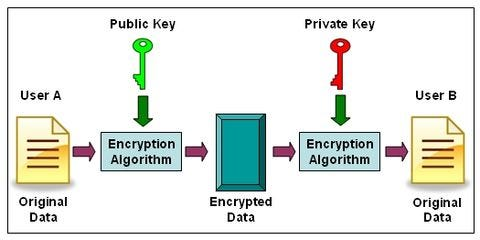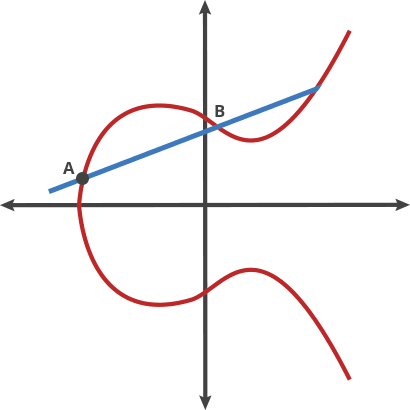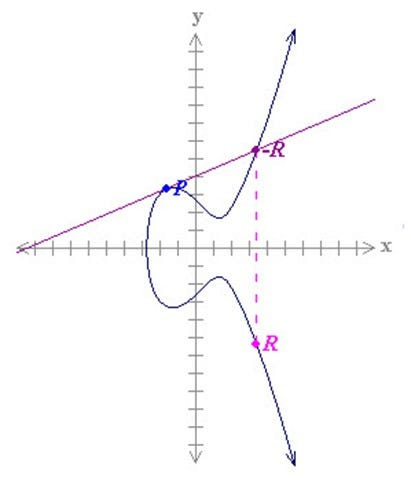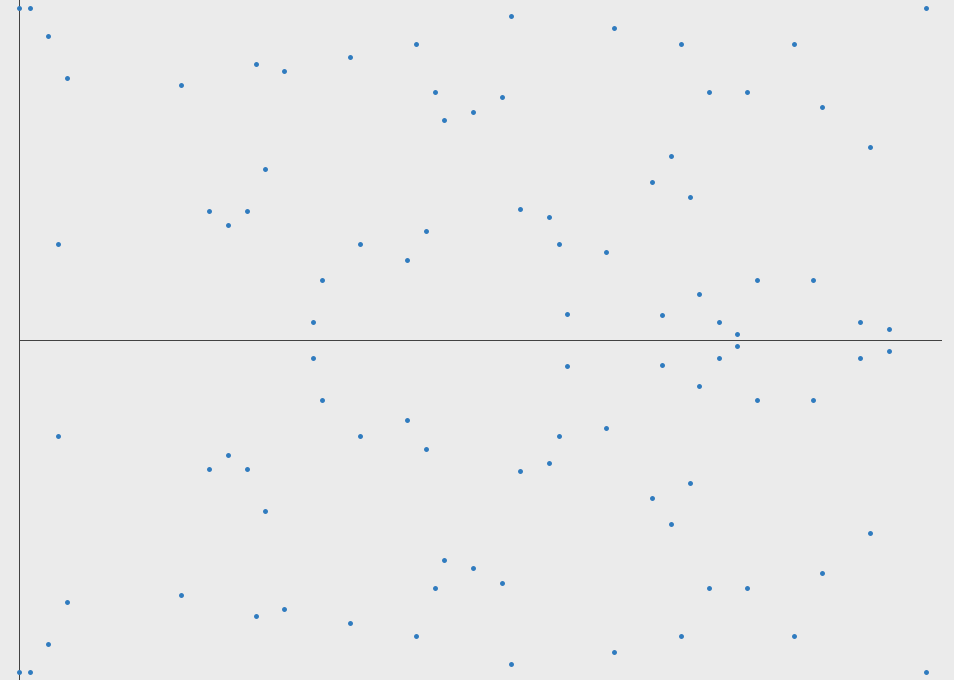# Basic Intro to Elliptic Curve Cryptography

Elliptic curve cryptography is a modern public-key encryption technique based on mathematical elliptic curves and is well-known for creating smaller, faster, and more efficient cryptographic keys. For example, Bitcoin uses ECC as its asymmetric cryptosystem because of its lightweight nature.

In this introduction to ECC, I want to focus on the high-level ideas that make ECC work. For the purposes of keeping this article easier to digest, I’ll omit implementation details and mathematical proofs, we can save those for another time.

## What is elliptic curve cryptography used for?

A common use of ECC is to encrypt data so that only authorized parties can decrypt it. This has several obvious use cases but is most often used to encrypt internet traffic. For example, on the Qvault web app I could used ECC to encrypt a verification email so that no one but the recipient can read the message.

## ECC is public-key cryptography

There are many types of public-key cryptography, and Elliptic Curve Cryptography is just one flavor. Other algorithms include RSA, Diffie-Helman, etc. Let’s go over a quick background of public-key cryptography as a jumping-off point, so that I can discuss ECC and build on top of these ideas. By all means, study more in-depth on public-key cryptography when you have the time.

Public-key cryptography allows the following to happen:wikia

We create two keys, a public key, and a private key. The public key is given freely, and any party can encrypt data by using it. However, the private key is kept secret and only those who hold it will have the ability to decrypt data.

## An example of public-key cryptography

Let’s pretend that Facebook is going to receive a private post from Donald Trump. Facebook needs to be able to ensure that when the ex-president sends his post over the internet, no one in the middle (Like the NSA, or an internet service provider) can read the message. The entire exchange using public-key cryptography would go like this:

• Donald Trump Notifies Facebook that he wants to send them a private post
• Facebook sends Donald Trump their public key
• Donald Trump uses the public key to encrypt his post:

“I love Fox and Friends” + Public Key = “s80s1s9sadjds9s”

• Donald Trump sends only the encrypted message to Facebook
• Facebook uses its private key to decrypt the message:

“s80s1s9sadjds9s” + Private Key = “I love Fox and Friends”

As you can see, this form of encryption can be quite useful. Here are some key points:

• The public key can safely be sent to anyone. It’s public.
• The private key must be kept safe because if someone in the middle were to get the private key, they could decrypt messages.
• Computers can quickly use the public key to encrypt a message, and quickly use the private key to decrypt a message.
• Computers require a very long time (millions of years) to derive the original data from the encrypted message if they don’t have the private key.

## How it Works: The Trapdoor Function

The crux of all public-key cryptographic algorithms is that they each have their own unique trapdoor function. A trapdoor function is a function that can only be computed one way, or at least can only be computed one way easily (in less than millions of years using modern computers).

### Not a trapdoor function:

`A + B = C`

If I’m given A and B I can compute C. However, if I’m given B and C I can also compute A. This is not a trapdoor function.

### Trapdoor function:

`"I love Fox and Friends” + Public Key --> s80s1s9sadjds9s`

If given “I love Fox and Friends” and the public key, I can produce `s80s1s9sadjds9s`, but if given `s80s1s9sadjds9s` and the Public Key I can’t produce “I love Fox and Friends”

In RSA, which is arguably the most widely used public-key cryptosystem, the trapdoor function relies on how hard it is to factor large numbers into their prime factors.

Public Key: `944,871,836,856,449,473`

Private Key: `961,748,941` and `982,451,653`

In the example above the public key is a very large number, and the private key is the two prime factors of the public key. This is a good example of a Trapdoor Function because it is very easy to multiply the numbers in the private key together to get the public key, but if all you have is the public key it will take a very long time using a computer to re-create the private key.

Note: In real cryptography, the private key would need to be 200+ digits long to be considered secure.

## What Makes Elliptic Curve Cryptography Different?

You would use ECC for the same reasons as RSA. ECC and RSA both generate a public and private key and allow two parties to communicate securely. One advantage to ECC however, is that a 256-bit key in ECC offers about the same security as a 3072-bit key using RSA. ECC allows resource-constrained systems like smartphones, embedded computers, and cryptocurrency networks to use ~10% of the storage space and bandwidth required by RSA.

## ECC’s Trapdoor Function

This is probably why most of you are here. The trapdoor function is what makes ECC special and different than RSA. The trapdoor function is similar to a mathematical game of pool.

First, we start with an arbitrary point on the curve. Next, we use the dot function to find a new point. Finally, we keep repeating the dot function to hop around the curve until we finally end up at our last point. Let’s walk through the algorithm.arstechnica
• Starting at `A`:
• `A dot B = -C` (Draw a line from A to B and it intersects at -C)
• Reflect across the X-axis from -C to C
• `A dot C = -D` (Draw a line from A to C and it intersects -D)
• Reflect across the X-axis from -D to D
• `A dot D = -E` (Draw a line from A to D and it intersects -E)
• Reflect across the X-axis from -E to E

This is a great trapdoor function because if you know where the starting point (A) is and how many hops are required to get to the ending point (E), it’s very easy to find the ending point. On the other hand, if all you know is where the starting point and ending point are, it’s nearly impossible to find how many hops it took to get there.

Public Key: Starting Point A, Ending Point E

Private Key: Number of hops from A to E

## Questions?

Here are a few questions I had when I first learned about ECC. Hopefully, I can address them properly.

### 1. How is the second point found? If the dot function is basically drawing a line between two points, don’t you need a second point to start with?

No. The second point (we will call it -R below) is actually the result of P dot P (let’s assume the first point is called P)

`P dot P = -R`

So what is `P dot P`? It is actually just the tangent line of P. See the graphic below:Image Source

### 2. What happens if the dot function produces a line that will go way off out to some extreme?

If the line doesn’t hit the curve close to the origin, we can actually define a maximum X value where the line will wrap back around and start from the beginning again. See the graphic below for an example.Image Source

### 3. If the number of hops is the private key, can’t I just count the hops until I hit the endpoint?

Nope! The number of hops is very large, something like `2^256`. It would take far too long to compute each hop one by one, for example `p dot p dot p dot p ...`.

If however, you know the number of hops you can use an exponentiation trick to find the ending point quite quickly. For example, and omitting the details of elliptic curve operations: `2P = P dot P` and then `4P = 2P dot 2P`. This allows you to get up to those crazy high calculations exponentially faster.

## Who Cares?

ECC is used as the cryptographic key algorithm in Bitcoin because it potentially can save ~90% of the resources used by a similar RSA system. It seems that each year we see more systems moving from RSA to a more modern elliptic curve approach.

## Thanks for reading, now take a course!

Interested in a high-paying job in tech? Land interviews and pass them with flying colors after taking my hands-on coding courses.

### Questions?

Follow and hit me up on Twitter @q_vault if you have any questions or comments. If I’ve made a mistake in the article be sure to let me know so I can get it corrected!

Subscribe to my newsletter for more coding articles delivered straight to your inbox.